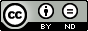### Regularization Method for Nonlinear Singularly Perturbed Systems of Fractional Order

Burkhan Kalimbetov, Riskeldy Turgunbaev

#### Abstract

The paper is devoted to construction of an asymptotic solution of a weakly nonlinear singularly perturbed differential system of fractional order. To construct the asymptotic solution we use ideas of normal differential forms method of V.F. Safonov. In the environment of the computer mathematical system Maple, approximate solutions are calculated, and corresponding solution schedules for various values of a small parameter are constructed.

#### Keywords

Nonlinear differential equation of fractional order, regularization, asymptotic, iteration problems, normal and unique solvability, computer mathematics system Maple.

PDF

#### References

Safonov, V.F. (1987) Multipoint resonance in a strongly nonlinear singularly perturbed system of differential equations. J. Differential Equations, 3, 529–530.

Safonov, V.F. (1989) Normal forms and regularization of nonlinear singularly perturbed evolution equations. J. Differential Equations, 25, 627–635.

Safonov, V.F. (1979) Regularization method for singularly perturbed systems of nonlinear differential equations. Izv. AS of USSR. Ser. mathem., 43, (3), 628–653.

Kalimbetov B.Т. (2007) Algorithm of method of normal forms for a weakly nonlinear equation with an unstable spectrum. Uzbek. Mathem. Journal, 2, 56–69.

Kalimbetov, B.Т. and Safonov, V.F. (1989) Normalized asymptotic solutions of weakly nonlinear singularly perturbed Cauchy problem with an unstable spectrum. Research on Integro-Differential. Equation, Frunze: "Ilim", 22, 37-42.

Dyakonov, V.P. Maple 7. Training Course. St. Petersburg, "Peter", 2003.

Kirsanov, M.N. Graphs in Maple. Moscow, Fizmathlit, 2007.

DOI: https://doi.org/10.24203/ajfam.v6i3.5598

### Refbacks

• There are currently no refbacks.

Copyright (c) 2018 Asian Journal of Fuzzy and Applied Mathematics